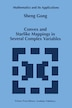Convex and Starlike Mappings in Several Complex Variables

bySheng Gong

Pricing and Purchase Info

\$137.95

Earn 690 plum® points

Prices and offers may vary in store

Quantity:

In stock online

Ships free on orders over \$25

Not available in stores

This interesting book deals with the theory of convex and starlike biholomorphic mappings in several complex variables. The underly­ ing theme is the extension to several complex variables of geometric aspects of the classical theory of univalent functions. Because the author's introduction provides an excellent overview of the content of the book, I will not duplicate the effort here. Rather, I will place the book into historical context. The theory of univalent functions long has been an important part of the study of holomorphic functions of one complex variable. The roots of the subject go back to the famous Riemann Mapping Theorem which asserts that a simply connected region n which is a proper subset of the complex plane C is biholomorphically equivalent to the open unit disk <. that="" _is2c_="" there="" is="" a="" univalent="" function="" _28_holoc2ad_="" morphic="" _bijection29_="" i="" _3a_=""><_20_-2b_20_n.20_in20_the20_early20_part20_of20_this20_century20_work20_began20_to20_focus20_on20_the20_class20_s20_of20_normalized20_28_f20_28_029_20_3d_20_020_and20_i27_27_20_28_029_20_3d_20_129_20_univalent20_functions20_defined20_on20_the20_unit20_disk.20_the20_restriction20_to20_unic2ad_20_valent20_functions20_defined20_on20_the20_unit20_disk20_is20_justified20_by20_the20_riemann20_mapping20_theorem.20_the20_subject20_contains20_many20_beautiful20_results20_that20_were20_obtained20_by20_fundamental20_techniques20_developed20_by20_many20_mathec2ad_20_maticians2c_20_including20_koebe2c_20_bieberbach2c_20_loewner2c_20_goluzin2c_20_grunsky2c_20_and20_schiffer.20_the20_best-known20_aspect20_of20_univalent20_function20_theory20_is20_the20_so-called20_bieberbach20_conjecture20_which20_was20_proved20_by20_de20_branges20_in20_1984. _-2b_="" n.="" in="" the="" early="" part="" of="" this="" century="" work="" began="" to="" focus="" on="" class="" s="" normalized="" _28_f="" _28_029_="1)" and="" _i27_27_="" univalent="" functions="" defined="" unit="" disk.="" restriction="" _unic2ad_="" valent="" disk="" is="" justified="" by="" riemann="" mapping="" theorem.="" subject="" contains="" many="" beautiful="" results="" that="" were="" obtained="" fundamental="" techniques="" developed="" _mathec2ad_="" _maticians2c_="" including="" _koebe2c_="" _bieberbach2c_="" _loewner2c_="" _goluzin2c_="" _grunsky2c_="" schiffer.="" best-known="" aspect="" function="" theory="" so-called="" bieberbach="" conjecture="" which="" was="" proved="" de="" branges="">
Title:Convex and Starlike Mappings in Several Complex VariablesFormat:PaperbackDimensions:209 pagesPublished:November 6, 2012Publisher:Springer-Verlag/Sci-Tech/TradeLanguage:English

The following ISBNs are associated with this title:

ISBN - 10:9401061912

ISBN - 13:9789401061919